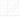# Minimum Time Visiting All Points

## Problem#

Given a `2D` plane (cartesian coordinate system), there are `n` points with integer coordinates `points[i] = [xi, yi]`. Our task is to find the minimum time in seconds to visit all points.

We can move according to the below rules:

• In `1` second, we can move either one unit vertically, one unit horizontally or diagonally (it means we move one unit vertically and one unit horizontally in one second).
• We have to visit the points in the same order as they appear in the array.
• We are allowed to pass through points that appear later in the order, but these do not count as visits.

## Input#Example 1:

```Input: points = [[1,1],[3,4],[-1,0]]
Output: 7
Explanation: One optimal path is [1,1] -> [2,2] -> [3,3] -> [3,4] -> [2,3] -> [1,2] -> [0,1] -> [-1,0]
Time from [1,1] to [3,4] = 3 seconds
Time from [3,4] to [-1,0] = 4 seconds
Total time = 7 seconds
```

Example 2:

```Input: points = [[3,2],[-2,2]]
Output: 5
```

Example 3:

```Input: points = [[3,2],[-2,-2]]
Output: 5
```

Constraints:

• `points.length == n`
• `1 <= n <= 100`
• `points[i].length == 2`
• `-1000 <= points[i], points[i] <= 1000`

## Solution#

### First Solution#

The optimal way to move from point `A` to point `B` is to move diagonally as a shortcut. The rest is to move in a straight line.

``````public int MinTimeToVisitAllPoints(int[][] points)
{
int min = 0, diffX = 0, diffY = 0;
for (int i = 0; i < points.Length - 1; i++)
{
diffX = Math.Abs(points[i + 1] - points[i]);
diffY = Math.Abs(points[i + 1] - points[i]);

min += (diffX < diffY) ? diffX : diffY;
min += Math.Abs(diffX - diffY);
}

return min;
}
``````

### Second Solution#

The second solution is slightly faster than the first solution because we use less arithmetic operation. The idea here is that when we add our `min` with `Math.Abs(diffX - diffY)` which is the rest straight line that we need to move, it is basically the same to finding the maximum different between `diffX` and `diffY`.

``````public int MinTimeToVisitAllPoints(int[][] points) {
int min = 0, diffX = 0, diffY = 0;
for (int i = 0; i < points.Length - 1; i++)
{
diffX = Math.Abs(points[i + 1] - points[i]);
diffY = Math.Abs(points[i + 1] - points[i]);

min += Math.Max(diffX, diffY);
}

return min;
}
``````

## Time Complexity#

The Time Complexity is O(n) because we have one loop where we iterate the input array which is `points`.

## Space Complexity#

The Time Complexity is O(1) because we don’t allocate memory with length `n` in our algorithm. We exclude array `points` because this is the input.